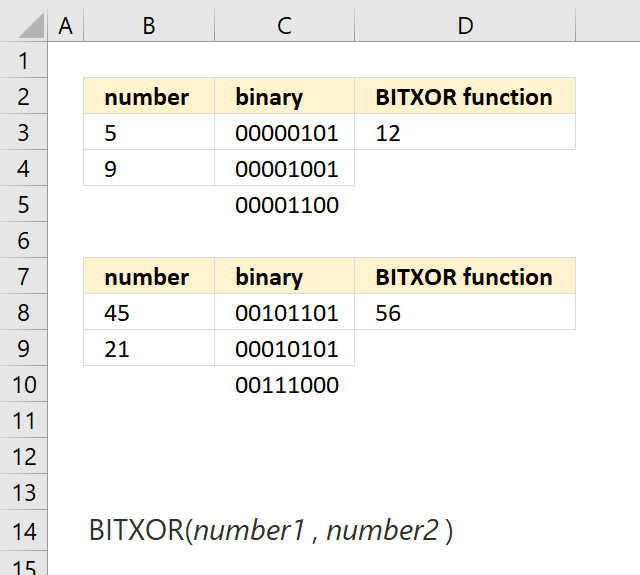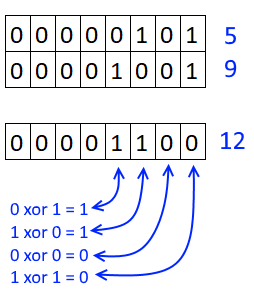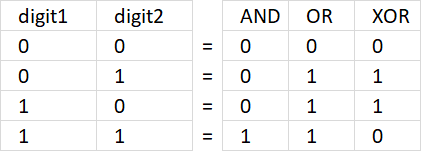Author: Oscar Cronquist Article last updated on May 11, 2022The BITXOR function calculates a decimal number that is a result of a bitwise comparison "XOR" of two numbers.

XOR is an abbreviation for "Exclusive OR" meaning if both digits at each position are not equal, 1 is returned for that position. If it is equal 0 (zero) is returned.

Formula in cell D3:

=BITXOR(B3, C3)

5 is 00000101 binary and 9 is 00001001. See picture below on how to do a bitwise "XOR".### Excel Function Syntax

BITXOR(number1number2)

### Arguments

 number1 Required. A number greater than 0 (zero). number2 Required. A number greater than 0 (zero).